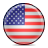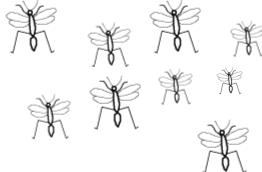# Would You Rather?

##### Age 7 to 11Challenge Level

If you are working in the USA units then go toWould you rather......

Have $10 \%$ of $£5$ or $75 \%$ of $80$p?Be given $60 \%$ of $2$ pizzas or $26 \%$ of $5$ pizzas?

Be bitten by $15 \%$ of $120$ mosquitoes or $8 \%$ of $250$ mosquitoes?Skip using a rope which is $54 \%$ of $105$cm long, or $88 \%$ of $2.75$m long?

Sit in a traffic jam for $33 \%$ of $2$ hours or $44 \%$ of $1$hr $40$mins?Make sure you explain your reasons clearly.

Perhaps you'd like to make up some of your own questions like these and send them to us. If you go to the solutions page you'll see some that have come in to us for you to have a look at and think about.